# 0708-1300/Class notes for Thursday, October 25

(diff) ← Older revision | Latest revision (diff) | Newer revision → (diff)
Jump to: navigation, search
Announcements go here

## Typed Notes

The notes below are by the students and for the students. Hopefully they are useful, but they come with no guarantee of any kind.

General Comments

1) Exam is on Nov 8th

2) Specs for the exam will be given next thursday.

Aside 2

Classification of Surfaces

1) Every 2 dimensional manifold that is compact connected and without boundary is smoothly equivalent to one of the following:

a) There is a family of such surfaces consisting of the sphere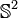$\mathbb{S}^2$, the torus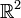$\mathbb{R}^2$ and various connected sums of many tori.

b) A connected sum of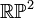$\mathbb{RP}^2$'s, the real projective plane. This is formed by taking the sphere$\mathbb{S}^2$ and identifying antipodal points.

Definition

A manifold without boundary has every point locally homeomorphic to a (relatively) open subset of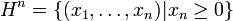$H^n=\{(x_1,\ldots,x_n)| x_n\geq 0\}$

2) If we now consider such manifolds WITH boundary they will be equivalent to something in case one only with open disks removed.

Aside 1

Classification of 1-manifolds

1) A compact 1-manifold without boundary is smoothly equivalent to a circle

2) A compact 1-manifold with boundary is smoothly equivalent to a closed interval

3) A non compact 1-manifold with boundary is smoothly equivalent to a half open interval

4) A non compact 1-manifold without boundary is smoothly equivalent to an open interval

Continuing with the Proof of the Whitney Embedding Theorem

We recall we are in part 3a of the proof.

The main steps we did in this last class were repeated again and so I won't do that here; however, there was an analogy to the proof presented that I will comment on.

Recall we have the idea of a remoteness function (that last class we called s) that we call r. If we were to consider the slice of the manifold with a certain value of r we will have problems with the fact that an embedding on one slice won't smoothly transition to an embedding on an adjacent slice. This problem is not solved from moving from a slice to disjoint intervals of values of r. However we get a nice analogy for this case:

We consider a roll of film on which each section (being the subset (not submanifold) of the manifold with values of r in some interval) occurring on each image in the roll of film. Thus as we move along the film we get to see the section with larger and larger r values. Indeed we could cut each image on the film up separately and lay them on top of each other in a stack. We would like this stack to correspond to an atlas. The problem of course is in the smoothness of the transition from one image to another.

The way we resolve this problem is to think of it as follows. We take an image and then shrink it down to zero and when we blow it back up again it is a new image. This is akin to turning the bulb off, then changing the film and then turning the bulb back on again.

In computer graphics, there is a problem where one tries to draw an image but that the processing time to draw the image is greater then the rate at which images are displayed. And hence part of the image is redrawn while part remains the same resulting in a distorted image. The solution is two have two buffers in memory. The image is displayed from one buffer while the next image is calculated and stored in the second buffer. Once the new image is completely drawn in it displayed on the screen and the original buffer is used to draw/compute the next image.

We now return to last class where we have defined the function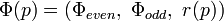$\Phi(p) = (\Phi_{even},\ \Phi_{odd},\ r(p))$ that went from the manifold into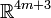$\mathbb{R}^{4m+3}$. (as each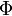$\Phi$ has dimension 2m+1 and an extra dimension for the r)

Here the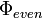$\Phi_{even}$ and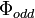$\Phi_{odd}$ correspond to the two buffers in the analogy.

Now this will turn out to be an embedding and we can reproduce our point on the manifold through use of the r. Indeed, the function is 1:1. Indeed for two points x ≠ y:

a) if we are near the same value of n then we are in the same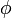$\phi$. Since each$\phi$ is an embedding (hence one-to-one)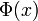$\Phi(x)$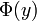$\Phi(y)$.

b) if we are not near the same n, then we will have different r's necessarily, so again$\Phi(x)$$\Phi(y)$.

Claim

A proper 1:1 continuous function is a homeomorphism onto its image.

i.e., we need to show a proper function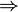$\Rightarrow$ closed.

Note

This claim shows that our map$\Phi$ is an embedding (provided such a proper r exists)

Summary of what is left to do:

1) Prove this claim

2) Prove the existence of such an r

3) Show how we can reduce from$\mathbb{R}^{4m+3}$ to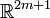$\mathbb{R}^{2m+1}$

Proof of 3

We can repeat the arguments used in part 2 of this proof again (the lack of compactness is not problem, as Sard's theorem and the dimension reducing argument doesn't depend on it)

However, we do have to make the following modification. In addition to not choosing a projection direction in im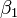$\beta_1$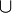$\cup$ im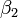$\beta_2$ we have to also make sure not to project in the vertical direction down into the r axis. But this is still quite possible by Sard's Theorem and hence we can reduce down to an embedding into$\mathbb{R}^{2m+1}$

Proof of 2

Consider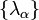$\{\lambda_{\alpha}\}$ a partition of unity subordinant to a countable cover by sets with compact closure. We can make this partition be countable as each chart with compact closure only needs finitely many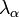$\lambda_{\alpha}$'s.

Hence we get a partition of unity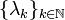$\{\lambda_k\}_{k\in\mathbb{N}}$ with supp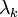$\lambda_k$ being compact.

Set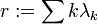$r:=\sum k\lambda_k$

Claim: r is proper

Proof'

Assume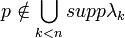$p\notin\bigcup_{k

then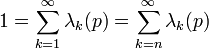$1=\sum_{k=1}^{\infty}\lambda_k (p) = \sum_{k=n}^{\infty}\lambda_k (p)$$\Rightarrow$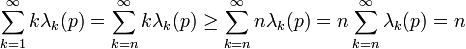$\sum_{k=1}^{\infty}k\lambda_k(p) =\sum_{k=n}^{\infty}k\lambda_k(p)\geq \sum_{k=n}^{\infty}n\lambda_k(p) = n\sum_{k=n}^{\infty}\lambda_k(p) =n$

so,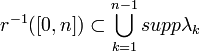$r^{-1}([0,n])\subset\bigcup_{k=1}^{n-1}supp\lambda_k$.

So compact

Q.E.D